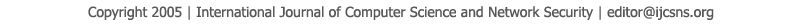To search, Click below search items.All Published Papers Search Service
 Title Author Year Keyword
 Title Wavelength Assignment Problem in Optical WDM Networks Author Amit Wason, R. S. Kaler Citation Vol. 7  No. 4  pp. 27-31 Abstract This paper proposes analyses of the wavelength assignment problem. The first-fit wavelength assignment algorithm is compared with the random wavelength assignment algorithm. These algorithms are compared on the basis of blocking probability; number of channels and number of links are kept constant whereas the response of the algorithms is calculated by varying the load per link (in Erlangs). The blocking probability is also calculated for the network with wavelength conversion and without wavelength conversion Keywords Wavelength division multiplexing (WDM), blocking probability, performance analyses, routing and wavelength assignment (RWA) URL http://paper.ijcsns.org/07_book/200704/20070404.pdf